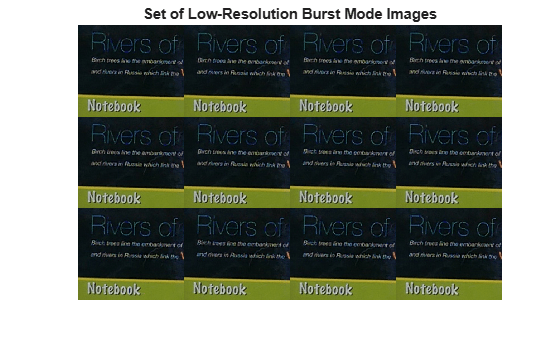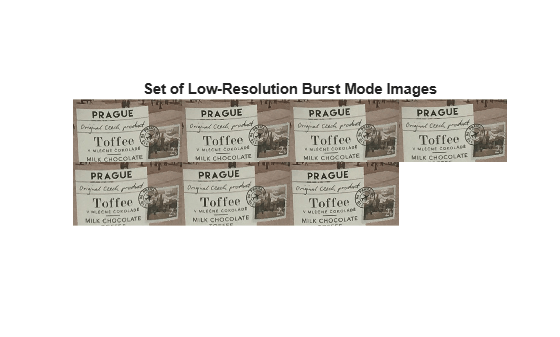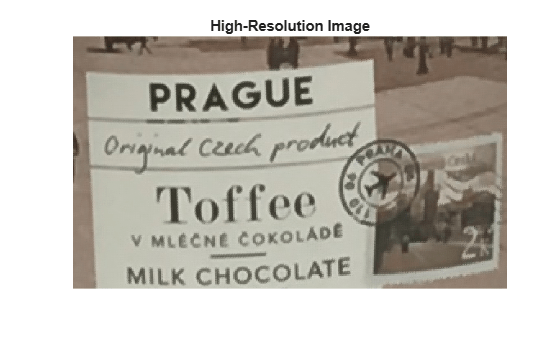burstinterpolant

Create high-resolution image from set of low-resolution burst mode images

Description

example

B = burstinterpolant(imds,tforms,scale) creates a high-resolution image, B from a set of low-resolution burst mode images stored as an ImageDatastore object, imds. scale specifies the magnification value for high-resolution image. The size of B is scale times the size of input images.

example

B = burstinterpolant(images,tforms,scale) creates a high-resolution image, B from a set of low-resolution burst mode images stored in cell array images. The size of B is scale times the size of input images.

Examples

collapse all

Specify the location of low-resolution burst mode images to be stored as an image datastore object. The input images are 2-D RGB images.

setDir = fullfile(toolboxdir('images'),'imdata','notebook');

Use the imageDatastore function to read and store the low-resolution burst mode images as an image datastore object.

imds = imageDatastore(setDir,'FileExtensions',{'.png'});

Display the images as a montage.

montage(imds)
title('Set of Low-Resolution Burst Mode Images')Compute Geometric Transformation Parameters

To compute geometric transformation parameters, convert all the RGB images into lightness images by using rgb2lightness function. The burst mode lightness images are stored as an image datastore object.

imdsTransformed = transform(imds,@(x) rgb2lightness(x));

Read the first lightness image into the workspace and use it as the reference image for estimating geometric transformations.

Get the optimal configuration parameters required for registration of the burst mode lightness images by using imregconfig function. Specify the image capture modality as 'monomodal'.

[optimizer,metric] = imregconfig('monomodal');

Find the total number of images stored in the image datastore object by using numpartitions function.

numImages = numpartitions(imds);

Create an array of 2-D affine transformation object to store 2-D affine transformations of each low-resolution burst mode lightness image excluding the reference image. Set the number of rows in the transformation array as total number of images in the image datastore object minus one.

tforms = repmat(affine2d(),numImages-1,1);

Use the imregtform function to estimate the rigid geometric transformations for each low-resolution burst mode lightness image with respect to the reference image.

idx = 1;
while hasdata(imdsTransformed)
tforms(idx) = imregtform(refImg,movingImg,'rigid',optimizer,metric);
idx = idx + 1;
end

Construct High-Resolution Image

Specify the scale factor for generating the high-resolution image.

scale = 4;

Create the high-resolution image from the set of low-resolution burst mode RGB images. Specify the transformation parameter to robustly estimate the high-resolution pixel values.

B = burstinterpolant(imds,tforms,scale);

Display the high-resolution image.

figure('WindowState','maximized')
imshow(B)
title ('High-Resolution Image')Read a low-resolution burst mode RGB image from the image datastore and display its size.

inputDim = [size(Img,1) size(Img,2)]
inputDim = 1×2

161   186

Display the size of the high-resolution image. Because the scale factor is 4, the size of the high-resolution image is 4 times the size of the low-resolution burst mode RGB images.

outputDim = [size(B,1) size(B,2)]
outputDim = 1×2

644   744

Load cell array data containing the low-resolution burst mode image into the workspace. The input images are monomodal and 2-D RGB images.

Display images in the cell array data as a montage.

montage(images,'Size',[2 4],'BackgroundColor',[1 1 1]);
title('Set of Low-Resolution Burst Mode Images')Compute Geometric Transformation Parameters

To compute geometric transformation parameters, convert all the RGB images into lightness images by using rgb2lightness function.

imagesT = cellfun(@rgb2lightness,images,'UniformOutput',false);

Read the first lightness image into the workspace and use it as the reference image for estimating geometric transformations.

refImg = imagesT{1};

Get the optimal configuration parameters required for registration of the burst mode lightness images by using imregconfig. Specify the image capture modality as 'monomodal'.

[optimizer,metric] = imregconfig('monomodal');

Find the total number of images stored in the cell array.

numImages = length(images);

Create an array of 2-D affine transformation object to store 2-D affine transformations of each low-resolution burst mode lightness image excluding the reference image. Set the number of rows in the transformation array as total number of images in the cell array minus one.

tforms = repmat(affine2d(),numImages-1,1);

Use the imregtform function to estimate the rigid geometric transformations for each low-resolution burst mode lightness image with respect to the reference image.

for i= 2:length(images)
movingImg = imagesT{i};
tforms(i-1) = imregtform(refImg,movingImg,'rigid',optimizer,metric);
end

Construct High-Resolution Image

Specify the scale factor for generating the high-resolution image.

scale = 3;

Construct the high-resolution image from the set of low-resolution burst mode RGB images. Specify the transformation parameter to robustly estimate the high-resolution pixel values.

B = burstinterpolant(images,tforms,scale);

Display the high-resolution image.

figure
imshow(B);
title ('High-Resolution Image')Read a low-resolution burst mode RGB image from the cell array and display its size.

Img = images{1};
inputDim = [size(Img,1) size(Img,2)]
inputDim = 1×2

154   265

Display the size of the high-resolution image. Because the scale factor is 3, the size of the high-resolution image is 3 times the size of the low-resolution burst mode images.

ouputDim = [size(B,1) size(B,2)]
ouputDim = 1×2

462   795

Input Arguments

collapse all

Input image datastore, specified as an ImageDatastore object. The input image datastore contains multiple low-resolution burst mode images used for creating the high-resolution image output.

• Images in the input image datastore must be 2-D grayscale images of size m-by-n or 2-D RGB images of size m-by-n-by-3.

• All images in the input image datastore must be of the same size and data type.

• The number of images in the input image datastore must be greater than or equal to 2.

Data Types: single | double | uint8 | uint16

Input images, specified as a k-by-1 cell array. k is the number of input images stored in the cell array. All the input images must have same size.

Data Types: single | double | uint8 | uint16

Transformation parameter, specified as an affine2d object array of size (k-1)-by-1 or 1-by-(k-1). k is the number of images in input imds or images.

Resize factor, specified as a scalar greater than or equal to 1.

Data Types: single | double | int8 | int16 | int32 | int64 | uint8 | uint16 | uint32 | uint64

Output Arguments

collapse all

High-resolution image, returned as a 2-D grayscale image or 2-D RGB image. B is of the same data type as the input images. The size of B is the value of scale times the size of the images in input imds or images.

For example, let L be the value of scale, and m-by-n be the size of the low-resolution burst mode images. Then, the size of the high-resolution image is mL-by-nL.

Tips

• Compute tforms with respect to each input image using the imregtform function. The first image in the input can be used as the reference image for estimating rigid geometric transformations (rotations and translations only).

• Compute input arguments optimizer and metric in imregtform using imregconfig function. optimizer must be a RegularStepGradientDescent object and metric must be a MeanSquares object.

• To improve the high-resolution output, you can modify the input argument value of RegularStepGradientDescent optimizer object in imregtform. For more details about these modifications, see the properties of RegularStepGradientDescent.

Algorithms

The burstinterpolant function uses the inverse distance weighting method  to generate high-resolution image from a set of low-resolution burst mode images. The function predicts a high-resolution pixel value from a set of pixels in the low-resolution burst mode images, selected based on the transformation parameter. The use of transformation parameter tforms makes the pixel selection robust to any rigid geometric transformations (rotations and translations only).

Note

• If the input images are 2-D RGB images, estimate tforms from the lightness component. You can use the rgb2lightness function to compute lightness values from the RGB color values.

 Shepard, Donald. “A Two-Dimensional Interpolation Function for Irregularly-Spaced Data”, In Proceedings of the 1968 23rd ACM National Conference, 517-524. New York, NY: ACM, 1968.